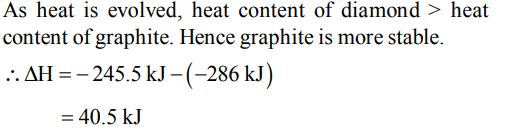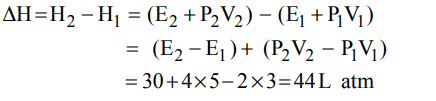## Thermodynamics Questions and Answers Part-2

1. Enthalpy of a reaction $\triangle H$ is expressed as
a) $\triangle H=\sum\triangle H_{p}-\sum\triangle H_{R}$
b) $\triangle H=\triangle H_{p}+\triangle H_{R}$
c) $\triangle H=\frac{\triangle H_{p}}{\triangle H_{R}}$
d) $\triangle H=\frac{\sum H_{p}}{\sum H_{R}}$

Explanation:2. The enthalpy change of a reaction does not depend on
a) The state of reactants and products
b) Nature of reactants and products
c) Different intermediate reactions
d) Initial and final enthalpy change of a reaction

Explanation: In accordance with Hess’s law

3. Enthalpy change $\left(\triangle H\right)$ of a system depends upon its
a) Initial state
b) Final state
c) Both on initial and final state
d) None of these

Explanation: Both on initial and final state

4. The relationship between enthalpy change and internal energy change is
a) $\triangle H=\triangle E+P\triangle V$
b) $\triangle H=\left(\triangle E+V\triangle P\right)$
c) $\triangle H=\triangle E-P\triangle V$
d) $\triangle H=V\triangle P -\triangle E$

Explanation: $\triangle$ H = $\triangle$ E + P $\triangle$ V

5. If a reaction involves only solids and liquids which of the following is true ?
a) $\triangle H<\triangle E$
b) $\triangle H=\triangle E$
c) $\triangle H>\triangle E$
d) $\triangle H=\triangle E+RT\triangle n$

Explanation: $\triangle$ H = $\triangle$ E + P $\triangle$ V, for solid and liquid, $\triangle$ V = 0 or $\triangle$ H = $\triangle$ E + $\triangle$ nRT, for solids and liquids $\triangle$ n = 0

6. $C\left(diamond\right)\rightarrow C\left(graphite\right),\triangle H$        = -ve. This shows that
a) Graphite is more stable
b) Graphite has more energy than diamond
c) Both are equally stable
d) Stability cannot be predicted

Explanation:7. One mole of a non-ideal gas undergoes a change of state $\left(2.0 atm, 3.0 L, 95 K\right)\rightarrow\left(4.0 atm, 5.0 L, 245 K\right)$         with a change in internal energy, $\triangle U= 30.0 L atm$    . The change in enthalpy $\triangle H$ of the process in L atm is.
a) 40.0
b) 42.3
c) 44.0
d) Not defined because pressure is not constant

Explanation:8. The enthalpy of vapourisation of water from the following two equations is.
$H_{2}\left(g\right)+\frac{1}{2}O_{2}\left(g\right)\rightarrow H_{2}O\left(l\right),\triangle$      H=-286kJ
$H_{2}\left(g\right)+\frac{1}{2}O_{2}\left(g\right)\rightarrow H_{2}O\left(g\right),\triangle$       H=-245.5kJ
a) 6.02 kJ
b) 40.5 kJ
c) 62.3 kJ
d) 1.25 kJ

Explanation:9. The variation of heat of reaction with temperature is given by:
a) Van’t Hoff equation
b) Clausius- Clapeyron equation
c) Nernst equation
d) Kirchoff’s equationa) $K=Ae^{-E_{a}/RT}$
b) $\log_{}{\frac{K_{2}}{K_{1}}}=\frac{E_{a}}{2.303R}\left(\frac{T_{2}-T_{1}}{T_{1}T_{2}}\right)$
c) $E_{CeII}=\frac{2.303RT}{nF}\log_{}{\frac{C_{2}}{C_{1}}}$
d) $\frac{\triangle H_{2}-\triangle H_{1} }{T_{2}-T_{1}}=\triangle C_{P}$
Explanation: $\frac{\triangle H_{2}-\triangle H_{1} }{T_{2}-T_{1}}=\triangle C_{P}$